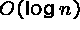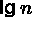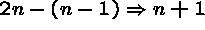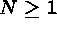Next: About this document Up: My Home Page

# Tree Structures Lecture 21

Steven S. Skiena

Trees

``I think that I shall never see a poem as lovely as a tree.
Poems are wrote by fools like me, but only G-d can make a tree.''
- Joyce Kilmer

We have seen many data structures which allow fast search, but not fast, flexible update.

Sorted Tables -search, O(n) insertion, O(n) deletion.

Hash Tables - The number of insertions are essentially bounded by the table size, which must be specified in advance. Worst case O(n) search.

Binary trees will enable us to search, insert, and delete fast, without predefining the size of our data structure!

How can we get this flexibility?

The only data structure we have seen which allows fast insertion/ deletion is the linked list, with updates in O(1) time but search in O(n) time.

To getsearch time, we used binary search, meaning we always had a choice of two next elements to look at.

To combine these ideas, we want a ``linked list'' with two pointers per node! This is the basic idea behind search trees!

Rooted Trees

We can use a recursive definition to specify what we mean by a ``rooted tree''.

A rooted tree is either (1) empty, or (2) consists of a node called the root, together with two rooted trees called the left subtree and right subtree of the root.

A binary tree is a rooted tree where each node has at most two descendants, the left child and the right child.

A binary tree can be implemented where each node has left and right pointer fields, an (optional) parent pointer, and a data field.

Rooted trees in Real Life

Rooted trees can be used to model corporate heirarchies and family trees.

Note the inherently recursive structure of rooted trees. Deleting the root gives rise to a certain number of smaller subtrees.

In a rooted tree, the order among ``brother'' nodes matters. Thus left is different from right. The five distinct binary trees with five nodes:

Binary Search Trees

A binary search tree is a binary tree where each node contains a key such that:

• All keys in the left subtree precede the key in the root.
• All keys in the right subtree succeed the key in the root.
• The left and right subtrees of the root are again binary search trees.

Left: A binary search tree. Right: A heap but not a binary search tree.

For any binary tree on n nodes, and any set of n keys, there is exactly one labeling to make it a binary search tree!!

Binary Tree Search

Searching a binary tree is almost like binary search! The difference is that instead of searching an array and defining the middle element ourselves, we just follow the appropriate pointer!

The type declaration is simply a linked list node with another pointer. Left and right pointers are identical types.

```TYPE
T = BRANDED REF RECORD
key: ElemT;
left, right: T := NIL;
END; (*T*)```

Dictionary search operations are easy in binary trees. The algorithm works because both the left and right subtrees of a binary search tree are binary search trees - recursive structure, recursive algorithm.

Search Implementation

```PROCEDURE Search(tree: T; e: ElemT): BOOLEAN =
(*Searches for an element e in tree.
Returns TRUE if present, else FALSE*)
BEGIN
IF tree = NIL THEN
RETURN FALSE                 (*not found*)
ELSIF tree.key = e THEN
RETURN TRUE                  (*found*)
ELSIF e < tree.key THEN
RETURN Search(tree.left, e)  (*search in left tree*)
ELSE
RETURN Search(tree.right, e) (*search in right tree*)
END; (*IF tree...*)
END Search;```

This takes time proportional to the height of the tree, O(h). Good, balanced trees have height, while bad, unbalanced trees have height O(n).

Building Binary Trees

To insert a new node into an existing tree, we search for where it should be, then replace that NIL pointer with a pointer to the new node.

Each NIL pointer defines a gap in the space of keys!

The pointer in the parent node must be modified to remember where we put the new node.

Insertion Routine

```PROCEDURE Insert(VAR tree: T; e: ElemT) =
BEGIN
IF tree = NIL THEN
tree:= NEW(T, key:= e);     (*insert at proper place*)
ELSIF e < tree.key THEN
Insert(tree.left, e)        (*search place in left tree*)
ELSE
Insert(tree.right, e)       (*search place in right tree*)
END; (*IF tree...*)
END Insert;```

Tree Shapes and Sizes

Suppose we have a binary tree with n nodes.

How many levels can it have? At leastand at most n.

How many pointers are in the tree? There are n nodes in tree, each of which has 2 pointers, for a total of 2n pointers regardless of shape.

How many pointers are NIL, i.e ``wasted''? Except for the root, each node in the tree is pointed to by one tree pointer Thus the number of NILs is, for.

Traversal of Binary Trees

How can we print out all the names in a family tree?

An essential component of many algorithms is to completely traverse a tree data structure. The key is to make sure we visit each node exactly once.

The order in which we explore each node and its children matters for many applications.

There are six permutations of {left, right, node} which define traversals. The most interesting traversals are inorder {left, node, right}, preorder {node, left, right}, postorder {left, right, node},

Why do we care about different traversals? Depending on what the tree represents, different traversals have different interpretations.

An in-order traversals of a binary serach tree sorts the keys!

Inorder traversal: 748251396, Preorder traversal: 124785369, Postorder traversal: 784529631

Reverse Polish notation is simply a post order traversal of an expression tree, like the one below for expression 2+3*4+(3*4)/5.

```  PROCEDURE Traverse(tree: T; action: Action;
order := Order.In; direction := Direction.Right) =

PROCEDURE PreL(x: T; depth: INTEGER) =
BEGIN
IF x # NIL THEN
action(x.key, depth);
PreL(x.left, depth + 1);
PreL(x.right, depth + 1);
END; (*IF x # NIL*)
END PreL;

PROCEDURE PreR(x: T; depth: INTEGER) =
BEGIN
IF x # NIL THEN
action(x.key, depth);
PreR(x.right, depth + 1);
PreR(x.left, depth + 1);
END; (*IF x # NIL*)
END PreR;

PROCEDURE InL(x: T; depth: INTEGER) =
BEGIN
IF x # NIL THEN
InL(x.left, depth + 1);
action(x.key, depth);
InL(x.right, depth + 1);
END; (*IF x # NIL*)
END InL;

PROCEDURE InR(x: T; depth: INTEGER) =
BEGIN
IF x # NIL THEN
InR(x.right, depth + 1);
action(x.key, depth);
InR(x.left, depth + 1);
END; (*IF x # NIL*)
END InR;

PROCEDURE PostL(x: T; depth: INTEGER) =
BEGIN
IF x # NIL THEN
PostL(x.left, depth + 1);
PostL(x.right, depth + 1);
action(x.key, depth);
END; (*IF x # NIL*)
END PostL;

PROCEDURE PostR(x: T; depth: INTEGER) =
BEGIN
IF x # NIL THEN
PostR(x.right, depth + 1);
PostR(x.left, depth + 1);
action(x.key, depth);
END; (*IF x # NIL*)
END PostR;

BEGIN (*Traverse*)
IF direction = Direction.Left THEN
CASE order OF
| Order.Pre   => PreL(tree, 0);
| Order.In => InL(tree, 0);
| Order.Post => PostL(tree, 0);
END (*CASE order*)
ELSE (* direction = Direction.Right*)
CASE order OF
| Order.Pre   => PreR(tree, 0);
| Order.In => InR(tree, 0);
| Order.Post => PostR(tree, 0);
END (*CASE order*)
END (*IF direction*)
END Traverse;```

Deletion from Binary Search Trees

Insertion was easy because the new node goes in as a leaf and only its parent is affected.

Deletion of a leaf is just as easy - set the parent pointer to NIL. But what if the node to be deleted is an interior node? We have two pointers to connect to only one parent!!

Deletion is somewhat more tricky than insertion, because the node to die may not be a leaf, and thus effect other nodes.

Case (a), where the node is a leaf, is simple - just NIL out the parents child pointer.

Case (b), where a node has one chld, the doomed node can just be cut out.

Case (c), relabel the node as its predecessor (which has at most one child when z has two children!) and delete the predecessor!

```  PROCEDURE Delete(VAR tree: T; e: ElemT): BOOLEAN =
(*Deletes an element e in tree.
Returns TRUE if present, else FALSE*)

PROCEDURE LeftLargest(VAR x: T) =
VAR y: T;
BEGIN
IF x.right = NIL THEN       (*x points to largest element left*)
y:= tree;                 (*y now points to target node*)
tree:= x;                 (*tree assumes the largest node to the left*)
x:= x.left;               (*Largest node left replaced by its left subtree*)
tree.left:= y.left;       (*tree assumes subtrees ...*)
tree.right:= y.right;     (*... of deleted node*)
ELSE                        (*Largest element left not found*)
LeftLargest(x.right)      (*Continue search to the right*)
END;
END LeftLargest;

BEGIN
IF tree = NIL      THEN RETURN FALSE
ELSIF e < tree.key THEN RETURN Delete(tree.left, e)
ELSIF e > tree.key THEN RETURN Delete(tree.right, e)
ELSE  (*found*)
IF tree.left = NIL THEN
tree:= tree.right;
ELSIF tree.right = NIL THEN
tree:= tree.left;
ELSE                        (*Target node has two nonempty subtrees*)
LeftLargest(tree.left)    (*Search in left subtree*)
END; (*IF tree.left...*)
RETURN TRUE
END; (*IF tree...*)
END Delete;```Next: About this document Up: My Home Page

Steve Skiena
Thu Nov 13 17:11:58 EST 1997